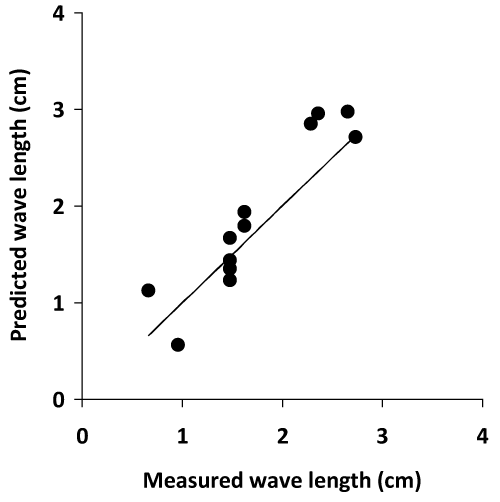Figure 14: Comparison of measured critical impulse wavelengths and values predicted using Equation (3), based on the physical dimensions of a single volume of abnormal, ischemic tissue. In the computational model the critical impulse wavelength, defining the abnormal physiology required for VT/VT, can be determined from the abnormal anatomy of the ischemic zone. The straight line at 45 degrees is the line of equality (y=x).